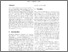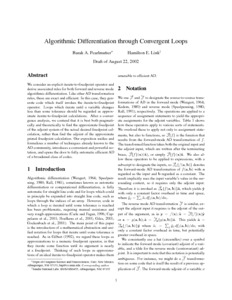# Algorithmic Differentiation through Convergent Loops

Pearlmutter, Barak A. and Link, Hamilton E. (2002) Algorithmic Differentiation through Convergent Loops. Project Report. UNSPECIFIED.Previewmore...Add this article to your Mendeley library

## Abstract

We consider an explicit iterate-to-fixedpoint operator and derive associated rules for both forward and reverse mode algorithmic differentiation. Like other AD transformation rules, these are exact and efficient. In this case, they generate code which itself invokes the iterate-to-fixedpoint operator. Loops which iterate until a variable changes less than some tolerance should be regarded as approximate iterate-to-fixedpoint calculations. After a convergence analysis, we contend that it is best both pragmatically and theoretically to find the approximate fixedpoint of the adjoint system of the actual desired fixedpoint calculation, rather than find the adjoint of the approximate primal fixedpoint calculation. Our exposition unifies and formalizes a number of techniques already known to the AD community, introduces a convenient and powerful notation, and opens the door to fully automatic efficient AD of a broadened class of codes.

Item Type: Monograph (Project Report) algorithmic differentiation; convergent loop; approximate fixedpoint; approximate primal fixedpoint calculation; variable change; iterate-to-fixedpoint operator; adjoint system; powerful notation; fixedpoint calculation ad transformation rule; exposition unifies; ad community mode algorithmic differentiation; broadened class; explicit iterate-to-fixedpoint operator; convergence analysis; automatic efficient ad; approximate iterate-to-fixedpoint calculation; Faculty of Science and Engineering > Computer Science 10237 Barak Pearlmutter 22 Nov 2018 14:44Item control page